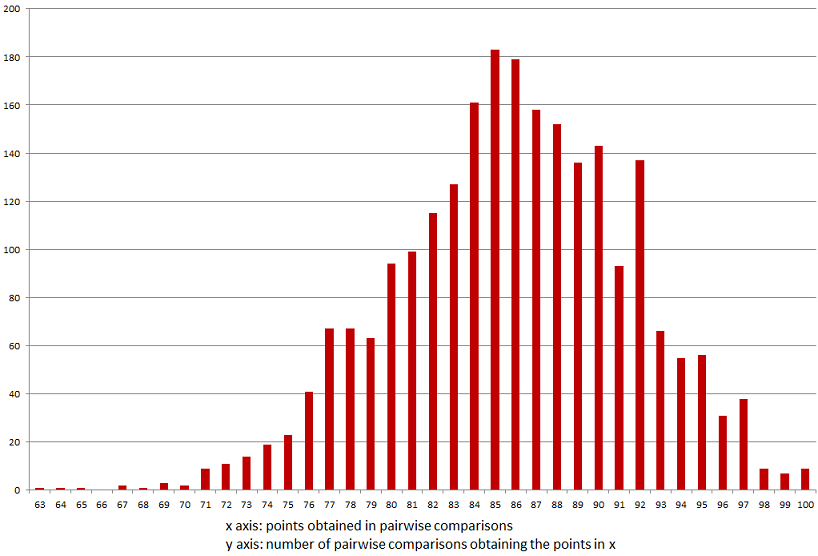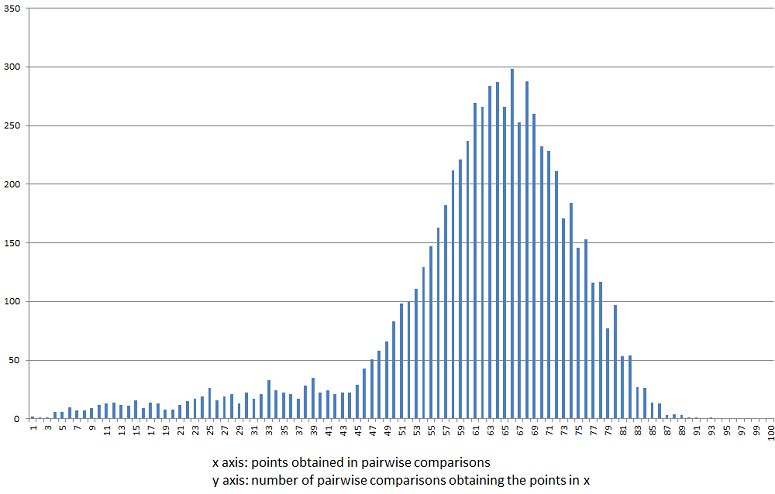### Challenging the proximity results using statistics

Statistics in elinguistics.net
Posted by: Vincent on Feb. 6, 2015, 20:10

## Statistical context - keeping statistical noise under control.

Whereas the results are convincing, there is a clear interference of chance which brings a 'statistic noise' in the output, most remarkably visible in mass comparisons. Whereas results bellow 67 to 69 seem to be very reliable, results above these distances are clearly influenced by chance. Without a clear vision about the impact of chance on the results, it is difficult to validate them: every discussion about a tree branch established by the system can run aground with the argumentation chance is the source of the data which represent a supposed relationship. Fine-tuning the results using statistics methods has two objectives:

1) Giving a solid basis to results by being able to quantify the probability that obtained genetic distances are due to chance (e.g. Russian to German have a genetic distance of 60,6, the probability of randomly getting this level of relatedness is lower than 0.01%. Mongolian and Manchu have a genetic distance of 79,46, the probability of getting a genetic distance lower or equal to 79,46 is 18.62%, so this last result is much less convincing than the first one). Assessing the average expected value and standard deviation will help. A first assessment on the basis of available data has been made (see further below).

2) Fine-tuning the results themselves: the number of consonants and the number of words used in the study is not constant. So each pairwise comparison has an own expected value and a specific expected standard deviation. Using a relation between the results of the pairwise comparisons and their respective expected values and expected standard deviations would help making results more resistant to statistical interference and thus explore more remote relationships between macro families of languages.

A glance at various result distributions gives a better understanding of the challenges of the use of statistics in elinguistics.net.

A distribution of randomly generated results gives an orientation of how reliable results of the study are. In elinguistics.net samples, we have 2773 pairwise comparisons of languages which are known as unrelated. Their mean, standard deviation and distribution give a good representation of what a mere random influence on elinguistics.net pairwise comparisons would be:- Distribution of 2773 random (not related) pairwise comparisons -

This is a data-centred assessment. The results range between 63 and 100 with an average of 81,74 and a standard deviation of 5,41. Three 'σ' are 16.23, which means that in elinguistics.net, results less than or equal to 66 (81,74-16,23=65,51) have a probability of only 0,4% to be due to chance! This assessment is based on results from all Indo-European languages compared to languages from all other families. So it is an observed result. The calculated average expected value is 83,36 with an average expected standard deviation of 5,03. The difference between the observed and calculated values can be explained with the fact that the system does identify clues to relationships between macro-families, so the observed results have a slightly lower average with a slightly higher standard deviation.

Results from pairwise comparisons within a genetically related group of languages bring a completely different distribution. Here is the distribution of results within the Indo-European macro family. The average result for 7021 pairwise comparisons is 60,34. Results range between 0 and 92.- Distribution of pairwise comparisons within the Indo-European macro family -

Bringing both distributions together gives a better understanding of the challenge (the red distribution has been scaled up with a factor of 7021/2773 to the blue one).The superposition illustrates perfectly the 'problem zone' of results between 66 and 86, which are shared by the influence of both genetic relationship and chance!

The results in eLinguistics are placed in their statistical context as the expected value (average distance if the comparaison is done with random results) and expected standard deviation are both specific to each comparison.

Blog author: